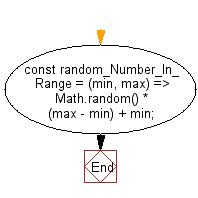# JavaScript: Get a random number in the specified range

## JavaScript fundamental (ES6 Syntax): Exercise-33 with Solution

Write a JavaScript program to get a random number in the specified range.

• Use Math.random() to generate a random value, map it to the desired range using multiplication.

Sample Solution:

JavaScript Code:

``````//#Source https://bit.ly/2neWfJ2
const random_Number_In_Range = (min, max) => Math.random() * (max - min) + min;
console.log(random_Number_In_Range(2, 10));
console.log(random_Number_In_Range(1, 5));
console.log(random_Number_In_Range(-5, -2));
console.log(random_Number_In_Range(0, 1));
```
```

Sample Output:

```5.897778190464821
1.1596294444724986
-2.644812110240778
0.5654096114937668
```

Pictorial Presentation:Flowchart:Live Demo:

See the Pen javascript-basic-exercise-1-33 by w3resource (@w3resource) on CodePen.

Improve this sample solution and post your code through Disqus

What is the difficulty level of this exercise?

Test your Programming skills with w3resource's quiz.

﻿

## JavaScript: Tips of the Day

Chunks an array into n smaller arrays

Example:

```const tips_chunkIntoN = (arr, n) => {
const size = Math.ceil(arr.length / n);
return Array.from({ length: n }, (v, i) =>
arr.slice(i * size, i * size + size)
);
}
console.log(tips_chunkIntoN([1, 2, 3, 4, 5, 6, 7,8], 4));
```

Output:

```[[1,2],[3,4],[5,6],[7,8]]
```